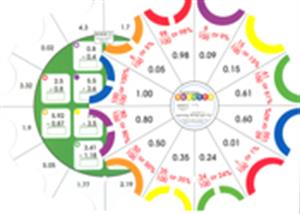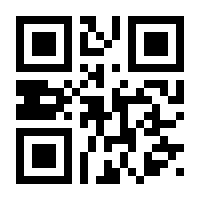See more:Most PopularMonthly Special

This set includes 144 challenges (12 cards with 12 challenges per card) giving students practice dealing with identification of decimals in tenths and hundredths, expressing decimals as fractions, comparing and ordering decimals, naming mixed decimals, identifying decimals along a number line, rounding decimals to the nearest whole number, adding and subtracting decimals and expressing decimals as fractions and percents.

This product meets the National Council of Teachers of Mathematics (NCTM) standards. Each card is SELF-CORRECTING.

These cards develop Numeration skills.

Card 1 -- Identify decimals as tenths.
Card 2 -- Express decimals (tenths) as fractions.
Card 3 -- Identify decimals as hundredths..
Card 4 -- Express decimals (tenths and hundredths) as fractions.
Card 5 -- Compare decimals.
Card 6 -- Order decimals.
Card 7 -- Name mixed decimals.
Card 8 -- Identify decimals on the number line.
Card 9 -- Round decimals (tenths) to the nearest whole number.
Card 11 -- Subtract decimals.
Card 12 -- Express decimals as fractions and percents.

To be used in conjunction with the "Learning Palette Base", part number LP-B100.This book contains scannable QR codes for additional features. See above description for details.
Price:
\$
9.99
\$
9.99
\$
Author:
Illustrator:
Series:
Learning Palette - Math
ISBN:
LP-M413
Binding:
Pages:
Size:
8 1/4 in diameter